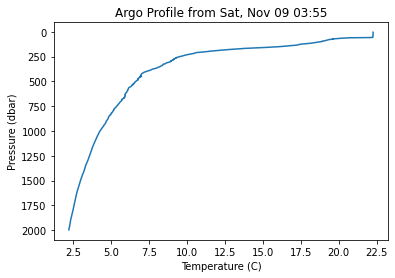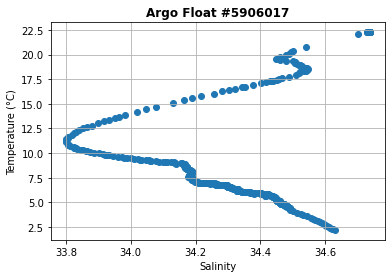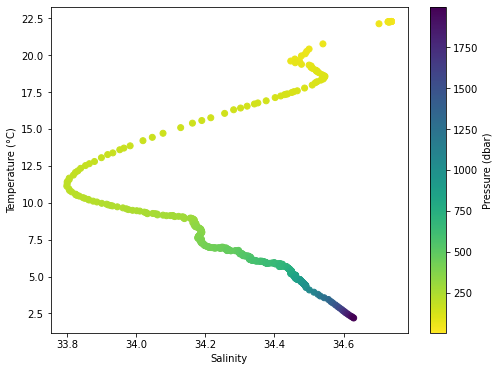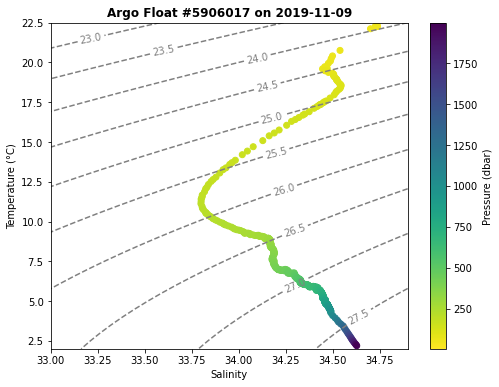# Introduction to Python – Argo Float Data

This summer, as part of our virtual REU workshop, we introduced students to the basics of using Python to analyze oceanographic data. When we frantically designed the course back in May (during the early even more frantic days of the pandemic), we originally intended to introduce students to data from both NDBC moorings and Argo profilers.  In the end, we only used NDBC, as that dataset was rich enough for students to work on a number of interesting research questions, while also learning python. Keeping things simple is a good mantra in both design and education.

But the Argo dataset, would have added another dimension to their learning, literally.I love the NDBC dataset, because it provides timeseries measurements of a few basic atmosphere/ocean variables at hundreds of locations all over the globe. It’s also relatively easy to access, and somewhat intuitive for students to understand, especially when they investigate data from stations near where they live.

But almost all of the data from NDBC is measured at or near the ocean surface.

What’s missing is depth, again literally.

That is why the Argo dataset is immensely useful. The Argo network now includes almost 4,000 real-time floats that students can use to investigate oceanic profiles from all over the world. They can look at timeseries of those profiles, or they can look at how profiles in a given area have changed over the past 20 years that the network has been active. Not only that, but a new set of Biogeochemical Argo floats is coming online, allowing students to study the subsurface structure of the ocean from several new instruments, with data that might help you enhance topics in chemical and biological oceanography courses.

Ultimately, this summer we decided that the NDBC dataset was more than enough to introduce students to the basics of python and timeseries data analysis, before they moved onto data from the OOI and other observatories for their research projects. The Argo dataset is a bit more complex to interpret, and that’s after you’ve figured out how to access that data you want. Because the network is a global partnership, there are a number of different data centers, each with its own subset of profilers and data format that takes some time to figure out.

But if you’re interested in in creating activities delve into the Argo dataset, hopefully you’ll find this notebook helpful as a first step.

This post is part of our 2020 Summer REU Intro to Python series. See also Part 1, Part 2, and Part 3.

2020 Data Labs REU

Written by Sage Lichtenwalner, Rutgers University, June 9, 2020

In this notebook we will cover some of basics of loading and plotting ARGO drifter in python.

Becuase this dataset inclues a depth dimension, this allows us to demonstrate several addtional graph types you will commonly see in oceanography, including profiles and TS diagrams.

In :
```# Notebook setup
import xarray as xr
!pip install netcdf4

import matplotlib.pyplot as plt
```
```Requirement already satisfied: netcdf4 in /usr/local/lib/python3.6/dist-packages (1.5.3)
Requirement already satisfied: cftime in /usr/local/lib/python3.6/dist-packages (from netcdf4) (1.1.3)
Requirement already satisfied: numpy>=1.7 in /usr/local/lib/python3.6/dist-packages (from netcdf4) (1.18.5)
```

# ARGO Drifter Data¶

While timeseries datasets at fixed points are very useful, the ocean is a deep place with a lot of interesting features and processes that vary with depth.

Thus, it's also important to be able to visualize data in ways that emphasize the depth dimension, just as we've emphasized the time dimension above.

To do so, we're going to load data from the Global Argo Float program, which had 3,962 active drifters around the world as of 6/6/2020.Unfortunately, because of the complexity and international nature of the program, there isn't one "perfect" source to retrieve Argo data, or even to search for drifters you may be interested in. However, I found the following sites helpful.

For the purposes of this activity, I picked an active drifter at random. Let's see what #5906017 can tell us about the ocean.

In :
```# Let's download a datafile to our serve (see the files tab)
```
```--2020-06-09 18:42:59--  https://data.nodc.noaa.gov/argo/gadr/data/aoml/5906017/nodc_5906017_prof.nc
Resolving data.nodc.noaa.gov (data.nodc.noaa.gov)... 140.90.235.40
Connecting to data.nodc.noaa.gov (data.nodc.noaa.gov)|140.90.235.40|:443... connected.
HTTP request sent, awaiting response... 200 OK
Length: 1167788 (1.1M) [application/x-netcdf]
Saving to: ‘nodc_5906017_prof.nc.2’

nodc_5906017_prof.n 100%[===================>]   1.11M   648KB/s    in 1.8s

2020-06-09 18:43:02 (648 KB/s) - ‘nodc_5906017_prof.nc.2’ saved [1167788/1167788]

```
In :
```# Load the dataset using xarray
data = xr.open_dataset('nodc_5906017_prof.nc')
data
```

As you can see, this dataset is a bit more complicated than our mooring timeseries dataset.

Argo uses the dimensions n_levels (for depth) and n_prof (for time).

In :
```# Quick Timeseries Profile plot of Temperature
plt.gca().invert_yaxis()
```Note, when possible, you should use the `_adjusted` variables, as those have been corrected. However, if you are interested in more recent data that hasn't been corrected yet, you will need to use the regular variable names (e.g. temp instead of temp_adjusted).

## Profile Plot¶

Now that we have the dataset loaded, let's create a profile plot of a single profile.

In :
```# Profile Plot
nprof = 25 #Specify a profile to plot

plt.xlabel('Temperature (C)')
plt.ylabel('Pressure (dbar)')
plt.title('Argo Profile from %s' % data.juld[nprof].dt.strftime('%a, %b %d %H:%M').values)

plt.gca().invert_yaxis() #Flip the y-axis
```Now let's get a little fancier and plot profiles of 2 variables.

In :
```# Profile Plot
# Subplot example
fig, (ax1,ax2) = plt.subplots(1,2, sharey=True, figsize=(10,6))

nprof = 0 # Fist profile

nprof = 25 # Middle-ish profile

nprof = -1 # Last profile

ax1.set_ylabel('Pressure (dbar)')
ax1.set_xlabel('Temperature (C)')
ax2.set_xlabel('Salinity')
ax1.invert_yaxis()
ax1.legend()

ax1.grid()
ax2.grid()

fig.suptitle('Argo Float #%d' % data.platform_number[nprof].values, fontweight='bold', fontsize=16);
```### Activity Break¶

Repeat the steps above to create profile plot of temperature and salinity using Float 5905077.

Note that this float has a lot of other variables that could be investigated.

In :
```# Your Turn - Download the data
```
In :
```# Your Turn - Load the data
```
In :
```# Your Turn - Profile plot
```

## T-S Diagram¶

Another popular plot in oceanography is the T-S Diagram, or Temperature-Salinity plot. It is commonly used to diagnose water masses in the global ocean, and to compare density stability of a water column profile (especially when lines of constant density are also included).

Basically, it's just a scatterplot of temp and salinity.

In :
```# TS Diagram
nprof = 25 #Selected profile
plt.xlabel('Salinity')
plt.ylabel('Temperature (°C)')
plt.grid()

plt.title('Argo Float #%d' % data.platform_number[nprof].values, fontweight='bold');
```That's nice, but we can also use a colored scatterplot to show the depth dimension.

In :
```# T-S Diagram with depth
plt.figure(figsize=(8,6))

nprof = 25 #Selected profile
plt.xlabel('Salinity');
plt.ylabel('Temperature (°C)')

cbh = plt.colorbar();
cbh.set_label('Pressure (dbar)')
```We used the default python colorbar for this plot (viridis), but there is a much larger colormap collection available.

With a little bit more code, we can add lines of constant density. This was adapted from the Ocean Python T-S Diagram example, but we will use meshgrid instead, since it makes the code a bit simpler.

To calculate density, we will need the wonderful seawater library.

In :
```!pip install seawater
import seawater
import numpy as np
```
```Requirement already satisfied: seawater in /usr/local/lib/python3.6/dist-packages (3.3.4)
Requirement already satisfied: numpy in /usr/local/lib/python3.6/dist-packages (from seawater) (1.18.5)
```
In :
```# TS Diagram with density contours
plt.figure(figsize=(8,6))

# Calculate the density lines
x = np.arange(33, 35, .1)
y = np.arange(2, 23, .5)
X, Y = np.meshgrid(x, y)
Z = seawater.eos80.dens0(X,Y) - 1000 # Substract 1000 to convert to sigma-t

# Plot the contour lines
CS = plt.contour(X, Y, Z, colors='grey', linestyles='dashed', levels=np.arange(22,30,.5))
plt.clabel(CS, inline=1, fontsize=10, fmt='%0.1f')

# Plot the data
nprof = 25 #Selected profile
plt.xlabel('Salinity');
plt.ylabel('Temperature (°C)')
plt.title('Argo Float #%d on %s' % (data.platform_number[nprof].values, data.juld[nprof].dt.strftime('%Y-%m-%d').values), fontweight='bold');

cbh = plt.colorbar(label='Pressure (dbar)');
```## Float Track Map¶

In :
```# Simple map of a float track
plt.figure(figsize=(8,6))
plt.plot(data.longitude, data.latitude, c='lightgrey')
plt.scatter(data.longitude, data.latitude, c=data.juld, cmap='RdYlBu')

# Crude profile labels
for jj in [1,25,-1]:
plt.text(data.longitude[jj]+.02, data.latitude[jj]+.02, data.n_prof[jj].values)

cbar = plt.colorbar();

# Fix the colorbar ticks
import pandas as pd # We need pandas for this
cbar.ax.set_yticklabels(pd.to_datetime(cbar.get_ticks()).strftime(date_format='%Y-%m-%d'));

# Set the aspect ratio to pseudo-Mercator
```## Fancier Maps with Cartopy¶

The cartopy library provides a lot of great features to create your own custom maps, though it takes a lot of patience to get it to work correctly.

In :
```# Install Cartopy in Google Colab
!apt-get -V -y -qq install python-cartopy python3-cartopy
!pip uninstall shapely -y
!pip install shapely --no-binary shapely
```
```Uninstalling Shapely-1.7.0:
Successfully uninstalled Shapely-1.7.0
Collecting shapely
Skipping wheel build for shapely, due to binaries being disabled for it.
ERROR: albumentations 0.1.12 has requirement imgaug<0.2.7,>=0.2.5, but you'll have imgaug 0.2.9 which is incompatible.
Installing collected packages: shapely
Running setup.py install for shapely ... done
Successfully installed shapely-1.7.0
```
In :
```import cartopy.crs as ccrs
import matplotlib.pyplot as plt
from cartopy.feature import NaturalEarthFeature
```
In :
```fig = plt.figure(figsize=(6.4,4.8),dpi=150)

ax = plt.axes(projection=ccrs.PlateCarree())
ax.set_extent([-130, -105, 22, 45])

# Basemaps
states = NaturalEarthFeature(category="cultural", scale="10m",
facecolor="none",
ax.coastlines('50m', linewidth=1)

ax.plot(data.longitude, data.latitude, linewidth=3, color='royalblue');
```For another example, check out this notebook which plots a glider track, colored by date, along with lat/lon labels and station locations.

Tags:
2 replies
1.Ajoy Kumar says:

Hi Sage: The part 4 of your phython programming does not work. I think the URL for the Argo’s data site is somehow mis-labelled. Thanks Ajoy

•Sage Lichtenwalner says:

Hi Ajoy, so it looks like NCEI has finally moved their data server for the data that used to be hosted by NODC. For whatever reason, the Argo data files aren’t on the new server, but hopefully they’ll add them back soon.

Alternatively, I recommend checking out the new argopy library, which I discovered after I wrote this tutorial. It makes searching and accessing Argo data in python far easier. You can also check out this recent paper by Maze and Balem in JOSS.

Hope that helps!